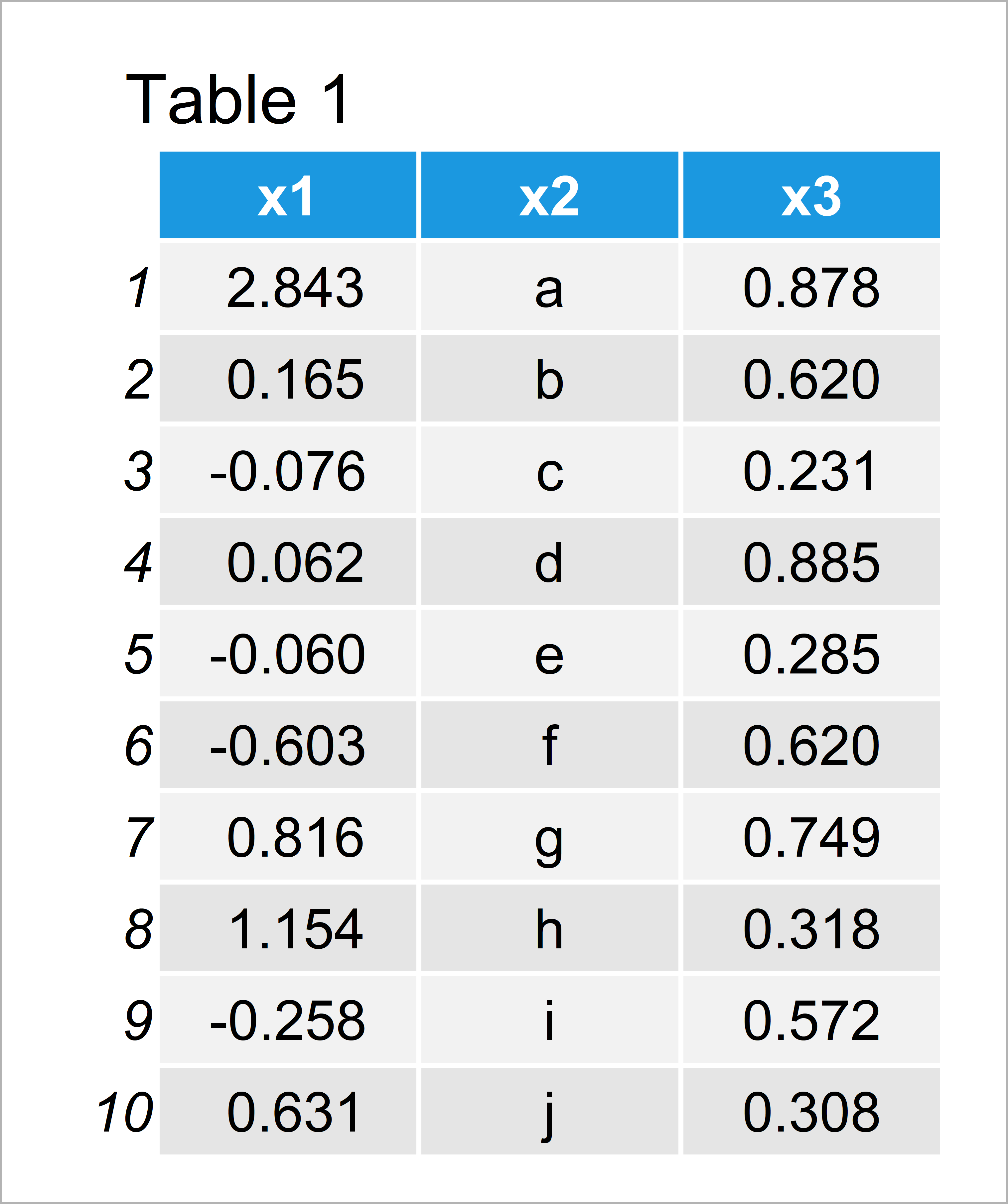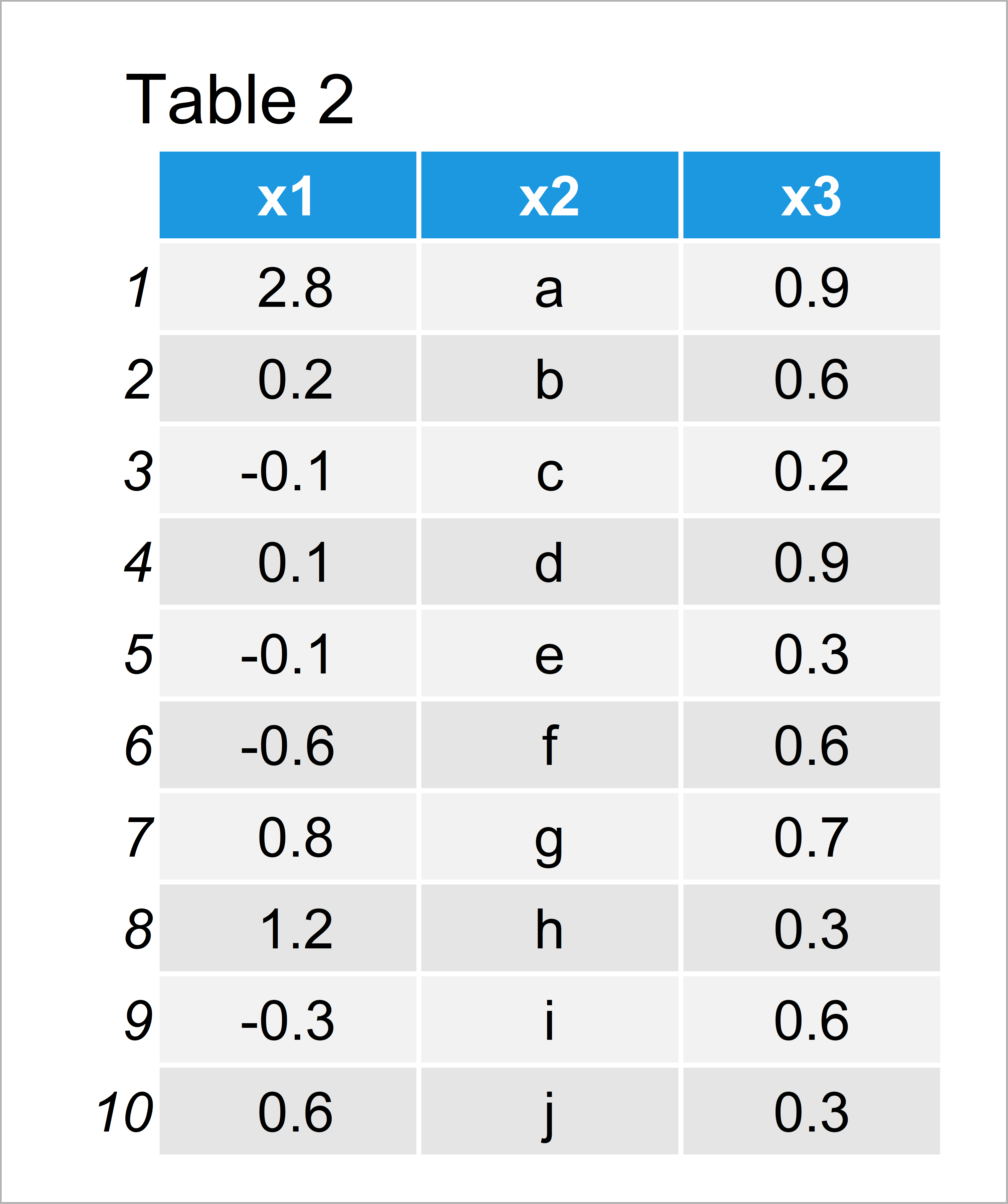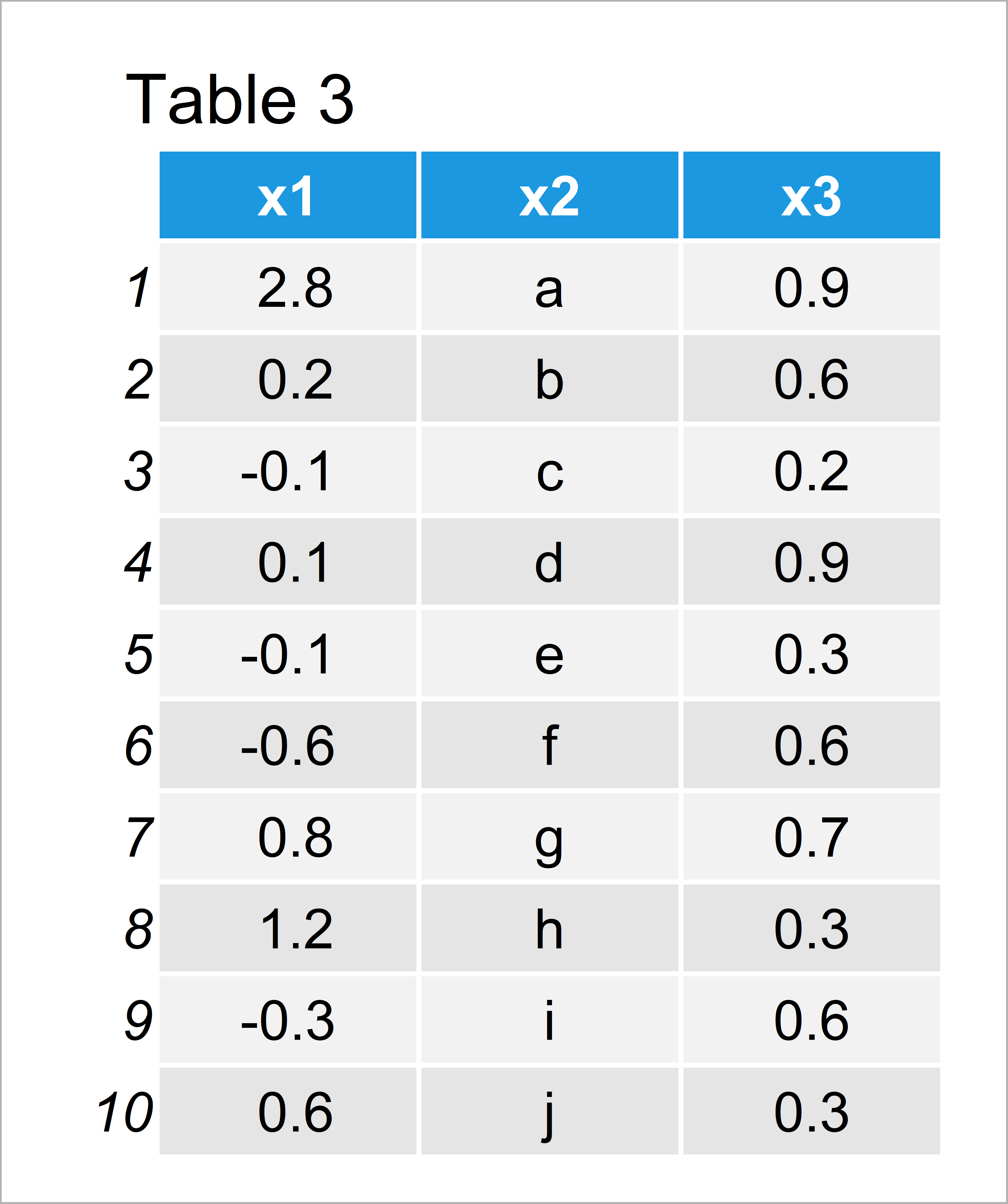# Round Numeric Columns of Data Frame with Character & Factor Variables in R (2 Examples)

In this article, I’ll explain how to round the digits of a data frame that contains not only numeric variables in the R programming language.

With that, let’s do this:

## Creation of Example Data

To begin with, we’ll have to create some data that we can use in the following example syntax:

```set.seed(65938)                           # Create example data frame
data <- data.frame(x1 = rnorm(10),
x2 = letters[1:10],
x3 = runif(10))
data                                      # Print example data frame```Table 1 shows that the example data has ten rows and three variables. The columns x1 and x3 are numeric and the column x2 has the character class.

## Example 1: Round Numeric Columns of Data Frame Using Base R

The following R programming syntax shows how to use the basic features of the R programming language to round numeric variables of our data frame.

In the following R syntax, we use a combination of the data.frame, lapply, function, if else, is.numeric, and round commands to round our numeric columns:

```data_round1 <- data.frame(lapply(data,    # Using Base R functions
function(x) if(is.numeric(x)) round(x, 1) else x))
data_round1                               # Print rounded data frame```As shown in Table 2, we have created a new data frame called data_round1 where the two numeric columns were rounded to 1 digit after the decimal point.

Note that we could round to another number of digits by changing the 1 within the round function of the previous code to any other number of digits we want.

As you have seen, it is possible to round exclusively the numeric values of a data frame using the basic installation of the R programming language.

However, this task can be done much easier… Let’s do this!

## Example 2: Round Numeric Columns of Data Frame Using dplyr Package

In Example 2, I’ll illustrate how to use the functions of the dplyr package to round each numeric variable of our data frame.

In order to use the functions of the dplyr package, we first need to install and load dplyr:

```install.packages("dplyr")                 # Install & load dplyr package
library("dplyr")```

Now, we can use the mutate_if function in combination with the is.numeric and round functions as shown below:

```data_round2 <- data %>%                   # Using dplyr functions
mutate_if(is.numeric,
round,
digits = 1)
data_round2                               # Print rounded data frame```Table 3 shows the output of the previous R syntax: It’s exactly the same data frame as in the previous example, but this time with much less complicated R syntax.

## Video, Further Resources & Summary

If you need further information on the contents of this tutorial, you might want to have a look at the following video of my YouTube channel. In the video, I’m explaining the topics of this tutorial.

Furthermore, you might have a look at the other articles on this website. Some articles are listed below.

In summary: In this article, I have explained how to round numeric variables of a mixed data frame in R programming. Don’t hesitate to let me know in the comments section, in case you have any additional questions.

Subscribe to the Statistics Globe Newsletter